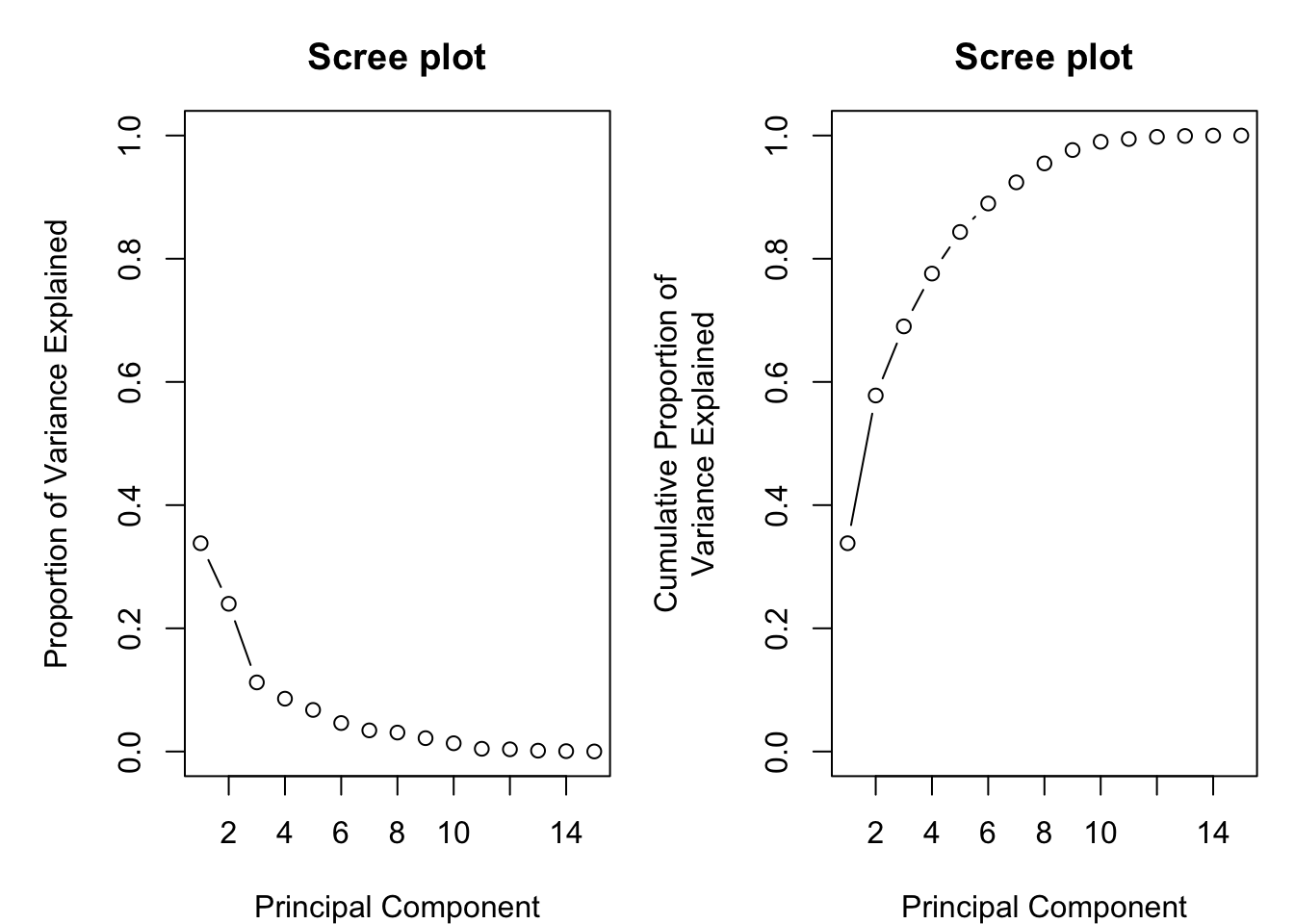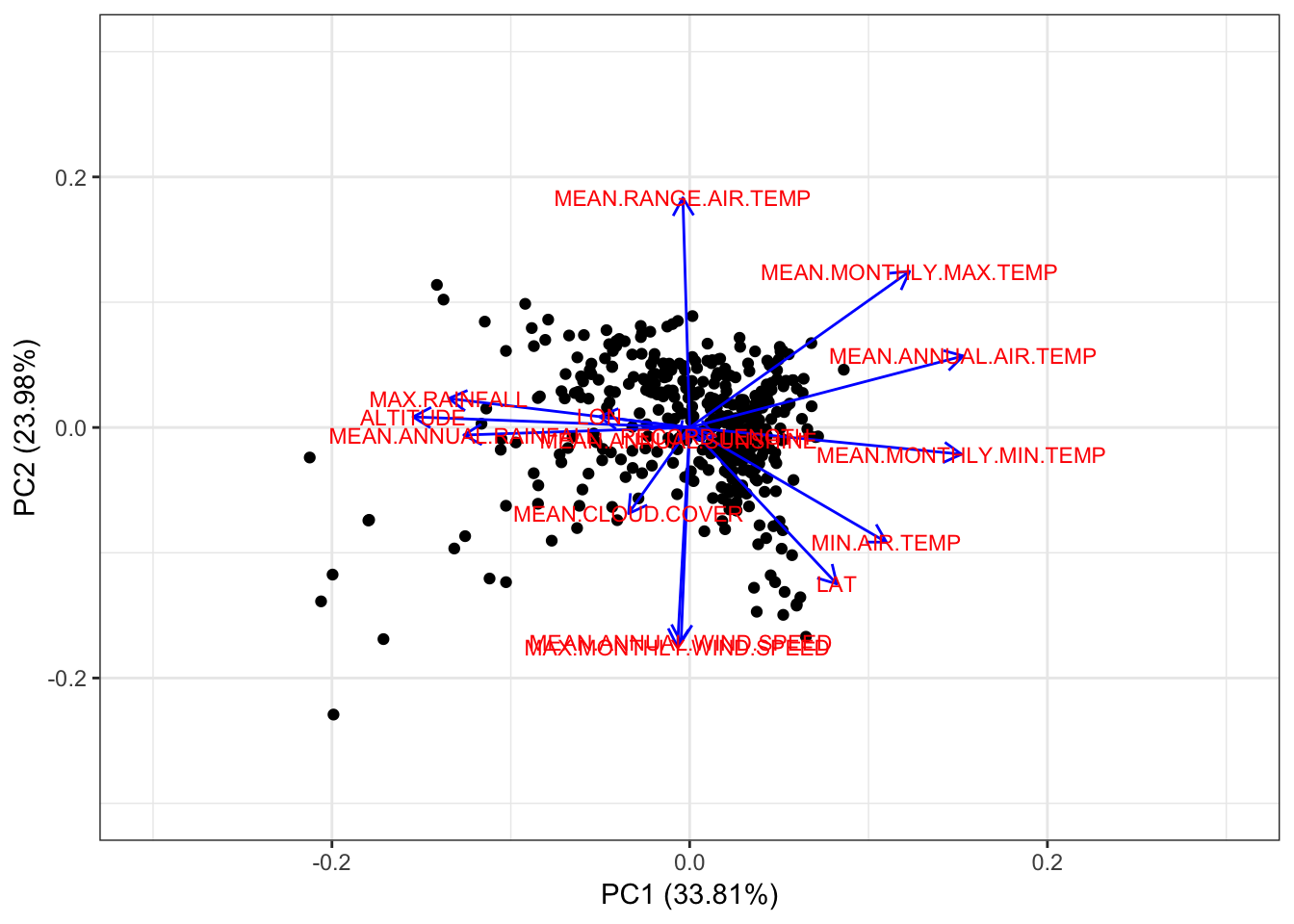Before we start with PCA we take a look at the mean and standard deviation of the variables in our data set.

``````# load data from previous sections
``apply(dwd.data.pca, 2, mean)``
``````##                    LAT                    LON               ALTITUDE
##              50.918438              10.200542             275.216625
##          RECORD.LENGTH   MEAN.ANNUAL.AIR.TEMP  MEAN.MONTHLY.MAX.TEMP
##              85.612091               8.406297              12.602519
##  MEAN.MONTHLY.MIN.TEMP MEAN.ANNUAL.WIND.SPEED       MEAN.CLOUD.COVER
##               4.531234               2.221662              67.128463
##   MEAN.ANNUAL.SUNSHINE   MEAN.ANNUAL.RAINFALL MAX.MONTHLY.WIND.SPEED
##            1514.627204             778.483627               2.798489
##           MAX.RAINFALL           MIN.AIR.TEMP    MEAN.RANGE.AIR.TEMP
##              38.443325             -14.784383               8.073048``````
``apply(dwd.data.pca, 2, sd)``
``````##                    LAT                    LON               ALTITUDE
##              1.9769500              2.1221118            273.5760257
##          RECORD.LENGTH   MEAN.ANNUAL.AIR.TEMP  MEAN.MONTHLY.MAX.TEMP
##             39.3965293              1.2476587              1.4562799
##  MEAN.MONTHLY.MIN.TEMP MEAN.ANNUAL.WIND.SPEED       MEAN.CLOUD.COVER
##              1.2089447              0.7115457              2.9739816
##   MEAN.ANNUAL.SUNSHINE   MEAN.ANNUAL.RAINFALL MAX.MONTHLY.WIND.SPEED
##            204.8230625            236.7348874              0.8100990
##           MAX.RAINFALL           MIN.AIR.TEMP    MEAN.RANGE.AIR.TEMP
##              7.6511660              2.5796323              1.1278853``````

We notice that the data is not on the same scale. Thus, when calling the `prcomp()` function we additionally center (`center = TRUE`) and standardize (`scale = TRUE`) the variables.

``pca <- prcomp(dwd.data.pca, center = TRUE, scale = TRUE)``

Let us check the dimensions of the principal component loading vectors and of the principal component scores.

``````# principal component loading vector
dim(pca\$rotation)``````
``##  15 15``
``````# principal component scores
dim(pca\$x)``````
``##  397  15``

All right, we got 397 observations and 15 principal components. In a next step we calculate the explained variance by each principal component.

``````pca.var = pca\$sdev^2
pca.ve = pca.var/sum(pca.var)
pca.ve``````
``````##   0.3381342639 0.2398253817 0.1121963410 0.0857383221 0.0675078494
##   0.0462335666 0.0343232615 0.0307857345 0.0216886543 0.0134126378
##  0.0044627818 0.0035870802 0.0013783619 0.0006104342 0.0001153292``````

We see that the first principal component accounts for 34% of the total variance in our data set, the second principal component for 24%, the third principal component for 11% and so forth. We plot the proportion of explained variance by each particular principal component with their absolute values (left plot) and as cumulative sums (right plot).

``````par(mfrow = c(1,2), mar = c(4,5,3,1))
plot(pca.ve,
xlab = "Principal Component",
ylab = "Proportion of Variance Explained",
ylim = c(0,1),
type = 'b',
main = 'Scree plot')

plot(cumsum(pca.ve),
xlab = "Principal Component",
ylab = "Cumulative Proportion of\nVariance Explained",
ylim = c(0,1),
type = 'b',
main = 'Scree plot')``````By eyeballing the scree plots above we see that after the third principal components there is drop off (elbow) of the proportion of variance explained. Moreover, the first three principal components account for approximately 69% of the explained variance in the data set. Based on these indicators it seems reasonable to keep the first three principal components to represent our data set.

``````pca.PC3 <- pca\$x[,1:3]

# save pca.PC3 for later usage
save(pca.PC3, file = 'pca_PC3_30300.RData')``````

Finally we take a look at the biplot. This time we plot the biplot by applying the `autoplot()` function from the `ggfortify` package, which allows more tweaking and results in more beautiful plots compared to the build-in `biplot()` function. We plot the combinations PC1 vs PC2 and PC1 vs PC3.

``````library(ggfortify)

x = 1, y = 2,
scale_x_continuous(limits = c(-0.3, 0.3))+
scale_y_continuous(limits = c(-0.3, 0.3))+
theme_bw()``````Recall how to interpret a biplot: The points show the observations in the plane formed by two principal components (here PC1 and PC2). We may look for patterns, cluster, and outliers. The arrows are vectors, corresponding to the original variables from which the principal components were computed. The orientation (direction/angle) of the vector is an indicator how much the variable contributes to the principal component PC space: the more parallel to a principal component axis, the more it contributes only to that PC. The length in the space of the vector indicates how much variability of this variable is represented by the two displayed principal components (here PC1 and PC2). The angles between vectors of different variables show their correlation in this space: small angles represent high positive correlation, right angles represent lack of correlation, opposite angles represent high negative correlation (Rossiter 2014).

Based on that reasoning and by examining the biplot above we may conclude that variables related to rainfall and the variable `ALTITUDE` are highly positive correlated and negatively correlated with variables related to temperatures. Further we realize that these vectors plot along the axis of the first principal component (PC1), thus indicating that PC1 accounts for the variability in the data set due to weather stations located in warm and dry low altitude regions and weather stations located in wet and cold high altitude regions. Similar conclusions can be drawn for the second principal component (PC2). PC2 accounts for the variability in the data set due to weather stations characterized by high wind speed and low seasonal variability in temperature, probably coastal regions, and weather stations characterized by low(er) wind speed and high(er) seasonal variability in temperature, probably regions with continental climates. Based on that characterization we may reference each particular point, and thus each particular weather station, and associate it with a particular domain. Let us consider the points that plot in the lower left quadrant; these are probably high altitude weather stations, characterized by low temperatures, high rainfall and gusty winds.

``````autoplot(pca,loadings = TRUE,
x = 1, y = 3,